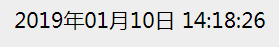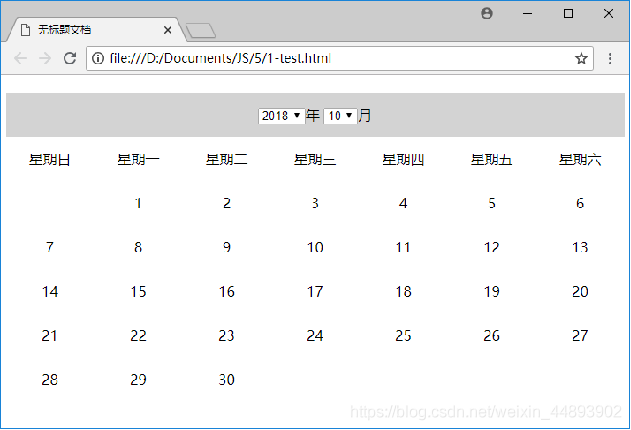# 从零开始学_JavaScript_系列（15）——js系列&lt;4&gt;（数值、字符串、对象、数组、函数、日期的基本方法）

<div id="a"></div>

<div id="a">内容</div>

（6）数值

①绝对值：Math.abc(x)         获得x的绝对值（之后的意思都类似，非特殊不再说明）

②求四舍五入后结果：

Math.round(1.9) = 2;

Math.round(1.1)=1;

③向上取整：

Math.ceil(1.1)=2;

Math.ceil(-1.1)=-1;

④向下取整：

Math.floor(1.9)=1;

Math.floor(-1.9)=-2;

⑤求最大值：

Math.max(1,2,3,4) = 4;

⑥求最小值

Math.min(-1,-2,-3,-4) = -4;

⑦随机数：

0 <= Math.random() < 1e指的是自然对数（以常数e为底数的对数叫做自然对数记作ln N(N>0).

⑨对字符串进行处理变为数字：

1            1

1.1        1

1.1a             1

1a11.1        1

a1.1             NaN

parseFloat("1.2a11.1")            1.2

Number(" 字符串 ")

1.1        1.1

1.1a             NaN

num.toFixed(digits);           输出的是 字符串

(100.111).toFixed(2); 输出是100.11

(100.1).toFixed(2)              输出的是100.10

(0).toFixed(2)                输出的是0.00

（7）字符串

str.length           返回字符串的长度（字符数）汉字是1个字符

②得到输入框（input）的长度：var str = input.value;

str.charAt(index)           得到字符串索引值（index）位置的字符

str.indexOf(字符串, 从哪个index开始找)          //第二个参数可以省略

str.search(regexp)              参数是正则表达式

var str="abc1";

$("#a").text(str.search(/[0-9]/)); 输出为3 var str="abc";$("#a").text(str.search(/[0-9]/));

str.match(regexp)  参数是正则表达式

varstr="abc1332d";

$("#a").text(str.match(/[0-9]/)); 返回值是1 例如：（加/g返回全部的） varstr="abc1332d";$("#a").text(str.match(/[0-9]/g));

str.replace(正则表达式|字符串被替换的字符串|函数)

var str="abc21";

$("#a").text(str.replace(/[0-9]/g,'q')); 返回值是：abcqq（两个数字都被替换为了q） 若不加g，那么则返回的是abcq1（第二个数字没有被替换） str.substring(索引1, 索引2) 返回索引1~索引2之间的字符串 前包含后不包含，例如： var str="abc21";$("#a").text(str.substring(1,2));

var str="abc21";

$("#a").text(str.substring(1)); 输出：bc21 str.slice(开始索引, 结束索引) 和上面功能一样，额外功能是，结束索引可以是负数。 假如是负数，那么从后往前数第n个，同样不包含结尾的。例如： var str="abc21";$("#a").text(str.slice(1,-1));

var str="abc21";

$("#a").text(str.slice(-1)); 输出1（因为从最后一个开始，到结尾，因此只有一个） str.substr(开始索引, 长度) 从开始输出，输出指定长度的 var str="abc21";$("#a").text(str.substr(1,2));

11str.split( 分隔符, 输出几个)          以分隔符为分割，输出数组

varstr="a1b1c1d";

$("#a").text(str.split("1")); 输出：数组a,b,c,d 分隔符也可以是正则表达式 var str="a1b1c1d";$("#a").text(str.split(/[0-9]/));

12str.toLowerCase()             将字符串变为小写

var str="ABC";

$("#a").text(str.toLowerCase()); 返回值是abc 13str.toUpperCase() 将字符串变大写 var str="aaaAs";$("#a").text(str.toUpperCase());

14String(被转对象)             转为字符串

var str = 111;

str = String(str);

$("#a").text(typeofstr); 返回值是：string 又例如： var str = true; str = String(str);$("#a").text(str);

true

str = String(str);

$("#a").text(str); 输出： function (){alert("1")} 15》转义字符 例如： var str ="a\"a";$("#a").text(str);

\"                        "

\\                         \

\t                         tab按键

（8）对象

①创建对象：

var obj = newOjbect{};

var obj = {};

②增加对象的方法：

ojb.name ="John";

③删除对象方法：

deleteobj.name;

④查看某个对象的类型：

obj.constructor;

var str ={};

str.name="John";

str.age=10;

if(str.constructor ==Object)

$("#a").text("111"); 是能输出111的 obj.toString() 将对象字符串（但并不是所有对象都可以） 例如： var str ={}; str.name="John"; str.age=10; var p = new Number(123);$("#a").text(p);

obj.valueOf()           获取某个对象的原始值

function abc(){this.a=1};

obj.hasOwnProperty(参数 )             返回参数是否存在于对象的属性中。

var str ={};

str.name="John";

str.age=10;

$("#a").text(str.hasOwnProperty("name")); 输出true （9）数组 ①创建数组： var array = new Array(); 或者是： var array =[]; 例：var array = [1, 3, 5]; 数组里可以是数字、字符串、对象、数组、bool值 以下假设数组为arr arr.length 数组的长度（空为0 arr[x] 数组下标为x的成员 ④修改数组元素，可以通过③中的直接修改。 arr.indexOf(元素, 从哪里索引开始) 第二个参数一般不用。返回值是索引值（和之前的indexOf用法一样）； arr.forEach(回调函数参数2) 遍历数组，回调函数遍历到每个成员都会执行。参数2可省略（貌似是什么this替代的什么的，不懂 回调函数的参数有三个：第一个是遍历到的元素，第二个是该元素的索引，第三个是这个数组本身。 例如：$(document).ready(function(){

var arr = [1, 3, 5 ,7];

var result = new String();

arr.forEach(function(item,index, array){

item += 1;

item =item.toString();

result += item+"的索引是" + index + "　";

})

$("#a").text(result); }); 输出是： 2的索引是0 4的索引是1 6的索引是2 8的索引是3 arr.reverse() 将数组的元素顺序颠倒一次 上代码即可：$(document).ready(function(){

var arr = [1, 3, 5 ,7];

var result = new String();

arr.reverse();

arr.forEach(function(item,index, array){

item =item.toString();

result += item+"的索引是" + index + "　";

})

$("#a").text(result); }); 输出： 7的索引是0 5的索引是1 3的索引是2 1的索引是3 arr.sort(回调函数 排序 不传回调函数的话，默认升序。 对字符串，按照Unicode码从第一个字符开始逐字符比较，然后排列。 例子：$(document).ready(function(){

var arr = [1, 3, 5, 7, 2,4, 6, 8];

arr.sort(function(a,b){

return b - a;            //b-a为降序，a-b为升序

});

$("#a").text(arr); }); 输出8,7,6,5,4,3,2,1 arr.push(元素1, 元素2, .....) 给已有数组加元素，加到最后位置。 arr.unshift( 元素1, 元素2, ...) 给已有数组添加，加到最前面 arr.shift() 获取数组第一个元素，并且从数组第一个元素中移除他。 如代码：$(document).ready(function(){

var arr = [1, 3, 5, 7, 2,4, 6, 8];

$("#a").text(arr); //从3开始输出 }); arr.pop() 类似上面，但从最后一个拿 11arr.splice( 从哪个索引开始删除多少, 插入的新元素); 可以用于添加、删除、替换等 var arr = [1, 3, 5, 7]; arr.splice(1,0,4); //位置1开始删除0个再添加4$("#a").text(arr);

var arr = [1, 3, 5, 7];

arr.splice(1,1);        //位置1删除1个于是剩下1,5,7

12arr.slice(开始索引, 结束索引)              返回值是数组的拷贝，开始位置包含结束不包含，注意这里没有p

var arr = [1, 3, 5, 7];

var b = arr.slice(1,2);          //从3开始5结束（不包含5）

$("#a").text(b); 输出3 第二个参数没有就表示到结束。 arr.slice(0); 效果是拷贝所有 13arr.concat( 1, 2, .....) 可以把几个数组连在一起，arr数组是第一个，值1是第二个。（注意，参数不一定是数组，也可以是其他类型） 例如： var arr = [1, 3, 5, 7]; var arr1 = [2,4,6]; var str = "aa"; var b = arr.concat(arr1,str);$("#a").text(b);

14arr.join( 分隔符 );

var arr = [1, 3, 5, 7];

arr = arr.join(" +");     //注意，这步的返回值才是我们想要的

$("#a").text(arr); 输出： 1 + 3 + 5 + 7 如果没有参数，则默认是逗号，如果参数是""，那么无分隔符 15arr.map( 回调函数 ) 遍历，并返回一个数组作为返回值 例如： var arr = [1, 3, 5, 7]; var arr1 =arr.map(function(item, index, array){ return item+1; });$("#a").text(arr1);

16arr.reduce( 回调函数, 传入的初始值begin )    具体要看回调函数

function( 上一次函数的返回值last, item, index, array){

retunr last + item;

}

last是上一次调用回调函数时的返回值，而第一次调用回调函数时，其值是begin。

var arr = [1, 3, 5, 7];

var arr1 =arr.reduce(function(last, item, index, array){

console.log(last);

return last + item;

}, 100);

$("#a").text(arr1); 输出值是116。原理是100 + 1 + 3 + 5 +7 console结果是100, 101， 104，109 注意：潜在的问题，没有执行第一次回调函数时的情况 假如没有给第二个参数begin，那么console.log只有三个结果（即1，4，9），说明第一次对于1，并没有执行回调函数。 如代码： var arr = [1, 3, 5, 7]; var arr1 =arr.reduce(function(last, item, index, array){ item += 1; return last + item; });$("#a").text(arr1);

（10）函数

var fun = function(a,b){return a+b}

fun(1)返回NaN——形参多

fun(2,3,4)返回5（因为2+3）——实参多

var arr = function(){

var total=0;

for(var i=0; i <arguments.length; i++)

{

total += arguments[i];

}

};

console.log(arr(1,2,3));

dog = {age:1};  //对象

var arr = function(obj){     //函数

obj.age++;

return obj;

};

cat = arr(dog);       //dog传递给函数，返回值给cat

console.log(dog.age); //dog被修改

cat.age++; //catdog对象的引用，修改属性

console.log(dog.age); //dog再次被修改

function 函数名( 形参 )

{

this.形参1 = 某个值;

this.函数内自定义变量名 = 某个值;

this.函数名 = function( 参数 )

{

函数内容

}

//这里可以理解为，隐含了一个return this

}

function fun( num ){ this.value = num;}

var obj1 = new fun(1);

console.log(obj1.value);

function fun( num ){

this.value = num;

}

fun.prototype.num=10;

fun.prototype.plus =function(){

this.value +=this.num;

}

var obj1 = new fun(1);

var obj2 = new fun(2);

console.log(obj2.num);     //输出10（说明和obj1互不干扰）

obj1.plus();

console.log(obj1.value);   //输出11

console.log(obj1.num);     //输出10

obj1.num++;   //10-》11

obj1.plus();      //11+11=22

console.log(obj1.value);   //输出22

console.log(obj1.num);     //输出11

（11）Date日期

①当前时间：new Date();

②指定年月日：new Date(年, 月-1,日, 时, 分, 秒);        ——参数是数字，

console.log(new Date(2016,4,4,1,2,3))

③获取某个具体的：（注意，date是在之前获取到的时间）

④时间格式化：

function dateFormat(date){

}

return num<10 ? "0" + num : num;

}

var date=new Date();

console.log(dateFormat(date));

2016-05-10 20:50:58

⑤设置date时间：

date.setFullYear(2010)   设置年份为2010

date.setDate(40)    会在当前月份基础上，查看第40天是哪天（下个月的哪天），即会自动递增。0 00 0js获取当前时间日期，并将其格式化为YYYY-MM-DD，并且自定义返回
js获取当前时间日期，并将其格式化为YYYY-MM-DD，并且自定义返回
0 0JS获取服务器日期，自动切换网页信息
JS获取服务器日期，自动切换网页信息
0 0JavaScript学习-日期对象、数组对象

0 00 0js实现回看近七天日期
js实现回看近七天日期
0 0JavaScript处理日期显示格式
JavaScript处理日期显示格式
0 00 0

JavaScript 自学手册文档教程311265

JavaScript入门与实战1889852

Javascript中的函数

Javascript异步编程

JS零基础入门教程（上册）